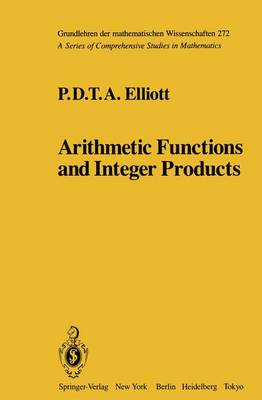•Arithmetic Functions and Integer Products - Grundlehren der mathematischen Wissenschaften 272 (Paperback)

(author)
£54.99
Paperback 461 Pages / Published: 18/10/2011
• We can order this

Usually dispatched within 3 weeks

Every positive integer m has a product representation of the form where v, k and the ni are positive integers, and each Ei = +/- I. A value can be given for v which is uniform in the m. A representation can be computed so that no ni exceeds a certain fixed power of 2m, and the number k of terms needed does not exceed a fixed power of log 2m. Consider next the collection of finite probability spaces whose associated measures assume only rational values. Let hex) be a real-valued function which measures the information in an event, depending only upon the probability x with which that event occurs. Assuming hex) to be non- negative, and to satisfy certain standard properties, it must have the form -A(x log x + (I - x) 10g(I -x". Except for a renormalization this is the well-known function of Shannon. What do these results have in common? They both apply the theory of arithmetic functions. The two widest classes of arithmetic functions are the real-valued additive and the complex-valued multiplicative functions. Beginning in the thirties of this century, the work of Erdos, Kac, Kubilius, Turan and others gave a discipline to the study of the general value distribution of arithmetic func- tions by the introduction of ideas, methods and results from the theory of Probability. I gave an account of the resulting extensive and still developing branch of Number Theory in volumes 239/240 of this series, under the title Probabilistic Number Theory.

Publisher: Springer-Verlag New York Inc.
ISBN: 9781461385509
Number of pages: 461
Weight: 727 g
Dimensions: 235 x 155 x 24 mm
Edition: Softcover reprint of the original 1st ed. 198